## Introducing cell interactions & transformations

### Introduction

PhysiCell 1.10.0 introduces a number of new features designed to simplify modeling of complex interactions and transformations. This blog post will introduce the underlying mathematics and show a complete example. We will teach this and other examples in greater depth at our upcoming Virtual PhysiCell Workshop & Hackathon (July 24-30, 2022). (Apply here!!)

### Cell Interactions

In the past, it has been possible to model complex cell-cell interactions (such as those needed for microecology and immunology) by connecting basic cell functions for interaction testing and custom functions. In particular, these functions were used for the COVID19 Coalition’s open source model of SARS-CoV-2 infections and immune responses. We now build upon that work to standardize and simplify these processes, making use of the cell’s state.neighbors (a list of all cells deemed to be within mechanical interaction distance).

All the parameters for cell-cell interactions are stored in cell.phenotype.cell_interactions.

#### Phagocytosis

A cell can phagocytose (ingest) another cell, with rates that vary with the cell’s live/dead status or the cell type. In particular, we store:

• double dead_phagocytosis_rate  : This is the rate at which a cell can phagocytose a dead cell of any type.
• std::vector<double> live_phagocytosis_rates : This is a vector of phagocytosis rates for live cells of specific types. If there are cell definitions in the simulation, then there are n rates in this vector. Please note that the index for any cell type is its index in the list of cell definitions, not necessarily the ID of its cell definition. For safety, use one of the following to determine that index:
• find_cell_definition_index( int type_ID ) : search for the cell definition’s index by its type ID (cell.type)
• find_cell_definition_index( std::string type_name ) : search for the cell definition’s index by its human-readable type name (cell.type_name)

We also supply a function to help access these live phagocytosis rates.

• double& live_phagocytosis_rate( std::string type_name )   : Directly access the cell’s rate of phagocytosing the live cell type by its human-readable name. For example,
pCell->phenotype.cell_interactions.live_phagocytosis_rate( "tumor cell" ) = 0.01;


At each mechanics time step (with duration $$\Delta t$$, default 0.1 minutes), each cell runs through its list of neighbors. If the neighbor is dead, its probability of phagocytosing it between $$t$$ and $$t+\Delta t$$ is

$\verb+dead_phagocytosis_rate+ \cdot \Delta t$

If the neighbor cell is alive and its type has index j, then its probability of phagocytosing the cell between $$t$$ and $$t+\Delta t$$ is

$\verb+live_phagocytosis_rates[j]+ \cdot \Delta t$

PhysiCell’s standardized phagocytosis model does the following:

• The phagocytosing cell will absorb the phagocytosed cell’s total solid volume into the cytoplasmic solid volume
• The phagocytosing cell will absorb the phagocytosed cell’s total fluid volume into the fluid volume
• The phagocytosing cell will absorb all of the phagocytosed cell’s internalized substrates.
• The phagocytosed cell is set to zero volume, flagged for removal, and set to not mechanically interact with remaining cells.
• The phagocytosing cell does not change its target volume. The standard volume model will gradually “digest” the absorbed volume as the cell shrinks towards its target volume.

#### Cell Attack

A cell can attack (cause damage to) another live cell, with rates that vary with the cell’s type. In particular, we store:

• double damage_rate  : This is the rate at which a cell causes (dimensionless) damage to a target cell. The cell’s total damage is stored in cell.state.damage.  The total integrated attack time is stored in cell.state.total_attack_time.
• std::vector<double> attack_rates : This is a vector of attack rates for live cells of specific types. If there are cell definitions in the simulation, then there are n rates in this vector. Please note that the index for any cell type is its index in the list of cell definitions, not necessarily the ID of its cell definition. For safety, use one of the following to determine that index:
• find_cell_definition_index( int type_ID ) : search for the cell definition’s index by its type ID (cell.type)
• find_cell_definition_index( std::string type_name ) : search for the cell definition’s index by its human-readable type name (cell.type_name)

We also supply a function to help access these attack rates.

• double& attack_rate( std::string type_name )   : Directly access the cell’s rate of attacking the live cell type by its human-readable name. For example,
pCell->phenotype.cell_interactions.attack_rate( "tumor cell" ) = 0.01;


At each mechanics time step (with duration $$\Delta t$$, default 0.1 minutes), each cell runs through its list of neighbors. If the neighbor cell is alive and its type has index j, then its probability of attacking the cell between $$t$$ and $$t+\Delta t$$ is

$\verb+attack_rates[j]+ \cdot \Delta t$

To attack the cell:

• The attacking cell will increase the target cell’s damage by $$\verb+damage_rate+ \cdot \Delta t$$.
• The attacking cell will increase the target cell’s total attack time by $$\Delta t$$.

Note that this allows us to have variable rates of damage (e.g., some immune cells may be “worn out”), and it allows us to distinguish between damage and integrated interaction time. (For example, you may want to write a model where damage can be repaired over time.)

As of this time, PhysiCell does not have a standardized model of death in response to damage. It is up to the modeler to vary a death rate with the total attack time or integrated damage, based on their hypotheses. For example:

$r_\textrm{apoptosis} = r_\textrm{apoptosis,0} + \left( r_\textrm{apoptosis,max} – r_\textrm{apoptosis,0} \right) \cdot \frac{ d^h }{ d_\textrm{halfmax}^h + d^h }$

In a phenotype function, we might write this as:

void damage_response( Cell* pCell, Phenotype& phenotype, double dt )
{ // get the base apoptosis rate from our cell definition
Cell_Definition* pCD = find_cell_definition( pCell->type_name );
double apoptosis_0 = pCD->phenotype.death.rates;
double apoptosis_max = 100 * apoptosis_0;
double half_max = 2.0;
double damage = pCell->state.damage;
phenotype.death.rates = apoptosis_0 +
(apoptosis_max -apoptosis_0) * Hill_response_function(d,half_max,1.0);
return;
}


#### Cell Fusion

A cell can fuse with another live cell, with rates that vary with the cell’s type. In particular, we store:

• std::vector<double> fusion_rates : This is a vector of fusion rates for live cells of specific types. If there are n cell definitions in the simulation, then there are n rates in this vector. Please note that the index i for any cell type is its index in the list of cell definitions, not necessarily the ID of its cell definition. For safety, use one of the following to determine that index:
• find_cell_definition_index( int type_ID ) : search for the cell definition’s index by its type ID (cell.type)
• find_cell_definition_index( std::string type_name ) : search for the cell definition’s index by its human-readable type name (cell.type_name)

We also supply a function to help access these fusion rates.

• double& fusion_rate( std::string type_name )   : Directly access the cell’s rate of fusing with the live cell type by its human-readable name. For example,
pCell->phenotype.cell_interactions.fusion_rate( "tumor cell" ) = 0.01;


At each mechanics time step (with duration $$\Delta t$$, default 0.1 minutes), each cell runs through its list of neighbors. If the neighbor cell is alive and its type has index j, then its probability of fusing with the cell between $$t$$ and $$t+\Delta t$$ is

$\verb+fusion_rates[j]+ \cdot \Delta t$

PhysiCell’s standardized fusion model does the following:

• The fusing cell will absorb the fused cell’s total cytoplasmic solid volume into the cytoplasmic solid volume
• The fusing cell will absorb the fused cell’s total nuclear volume into the nuclear solid volume
• The fusing cell will keep track of its number of nuclei in state.number_of_nuclei.  (So, if the fusing cell has 3 nuclei and the fused cell has 2 nuclei, then the new cell will have 5 nuclei.)
• The fusing cell will absorb the fused cell’s total fluid volume into the fluid volume
• The fusing cell will absorb all of the fused cell’s internalized substrates.
• The fused cell is set to zero volume, flagged for removal, and set to not mechanically interact with remaining cells.
• The fused cell will be moved to the (volume weighted) center of mass of the two original cells.
• The fused cell does change its target volume to the sum of the original two cells’ target volumes. The combined cell will grow / shrink towards its new target volume.

### Cell Transformations

A live cell can transform to another type (e.g., via differentiation). We store parameters for cell transformations in cell.phenotype.cell_transformations, particularly:

• std::vector<double> transformation_rates : This is a vector of transformation rates from a cell’s current to type to one of the other cell types. If there are cell definitions in the simulation, then there are n rates in this vector. Please note that the index for any cell type is its index in the list of cell definitions, not necessarily the ID of its cell definition. For safety, use one of the following to determine that index:
• find_cell_definition_index( int type_ID ) : search for the cell definition’s index by its type ID (cell.type)
• find_cell_definition_index( std::string type_name ) : search for the cell definition’s index by its human-readable type name (cell.type_name)

We also supply a function to help access these transformation rates.

• double& transformation_rate( std::string type_name )   : Directly access the cell’s rate of transforming into a specific cell type by searching for its human-readable name. For example,
pCell->phenotype.cell_transformations.transformation_rate( "mutant tumor cell" ) = 0.01;


At each phenotype time step (with duration $$\Delta t$$, default 6 minutes), each cell runs through the vector of transformation rates. If a (different) cell type has index j, then the cell’s probability transforming to that type between $$t$$ and $$t+\Delta t$$ is

$\verb+transformation_rates[j]+ \cdot \Delta t$

PhysiCell uses the built-in Cell::convert_to_cell_definition( Cell_Definition& ) for the transformation.

### Other New Features

This release also includes a number of handy new features:

After setting chemotaxis sensitivities in phenotype.motility and enabling the feature, cells can chemotax based on linear combinations of substrate gradients.

• std::vector<double> phenotype.motility.chemotactic_sensitivities is a vector of chemotactic sensitivities, one for each substrate in the environment. By default, these are all zero for backwards compatibility. A positive sensitivity denotes chemotaxis up a corresponding substrate’s gradient (towards higher values), whereas a negative sensitivity gives chemotaxis against a gradient (towards lower values).
• For convenience, you can access (read and write) a substrate’s chemotactic sensitivity via phenotype.motility.chemotactic_sensitivity(name) where name is the human-readable name of a substrate in the simulation.
• If the user sets cell.cell_functions.update_migration_bias = advanced_chemotaxis_function, then these sensitivities are used to set the migration bias direction via: $\vec{d}_\textrm{mot} = s_0 \nabla \rho_0 + \cdots + s_n \nabla \rho_n.$
• If the user sets cell.cell_functions.update_migration_bias = advanced_chemotaxis_function_normalized, then these sensitivities are used to set the migration bias direction via: $\vec{d}_\textrm{mot} = s_0 \frac{\nabla\rho_0}{|\nabla\rho_0|} + \cdots + s_n \frac{ \nabla \rho_n }{|\nabla\rho_n|}.$

We now allow more nuanced, type-specific adhesion strengths to allow differential adhesion (e.g,. heterotypic versus homotypic).
• cell.phenotype.mechanics.adhesion_affinities is a vector of adhesive affinities, one for each cell type in the simulation. By default, these are all one for backwards compatibility.
• For convenience, you can access (read and write) a cell’s adhesive affinity for a specific cell type via phenotype.mechanics.adhesive_affinity(name), where name is the human-readable name of a cell type in the simulation.
• The standard mechanics function (based on potentials) uses this as follows. If cell i has an cell-cell adhesion strength a_i and an adhesive affinity p_ij to cell type j , and if cell j has a cell-cell adhesion strength of a_j and an adhesive affinity p_ji to cell type i, then the strength of their adhesion is $\sqrt{ a_i p_{ij} \cdot a_j p_{ji} }.$ Notice that if $$a_i = a_j$$ and $$p_{ij} = p_{ji}$$, then this reduces to $$a_i a_{pj}$$.
• The standard elastic spring function (standard_elastic_contact_function) uses this as follows. If cell i has an elastic constant a_i and an adhesive affinity p_ij to cell type j , and if cell j has an elastic constant a_j and an adhesive affinity p_ji to cell type i, then the strength of their adhesion is $\sqrt{ a_i p_{ij} \cdot a_j p_{ji} }.$ Notice that if $$a_i = a_j$$ and $$p_{ij} = p_{ji}$$, then this reduces to $$a_i a_{pj}$$.

### A brief immunology example

We will illustrate the major new functions with a simplified cancer-immune model, where tumor cells attract macrophages, which in turn attract effector T cells to damage and kill tumor cells. A mutation process will transform WT cancer cells into mutant cells that can better evade this immune response.

#### The problem:

We will build a simplified cancer-immune system to illustrate the new behaviors.

• WT cancer cells: Proliferate and release a signal that will stimulate an immune response. Cancer cells can transform into mutant cancer cells. Dead cells release debris. Damage increases apoptotic cell death.
• Mutant cancer cells: Proliferate but release less of the signal, as a model of immune evasion. Dead cells release debris. Damage increases apoptotic cell death.
• Macrophages: Chemotax towards the tumor signal and debris, and release a pro-inflammatory signal. They phagocytose dead cells.
• CD8+ T cells: Chemotax towards the pro-inflammatory signal and cause damage to tumor cells. As a model of immune evasion, CD8+ T cells can damage WT cancer cells faster than mutant cancer cells.

We will use the following diffusing factors:

• Tumor signal: Released by WT and mutant cells at different rates. We’ll fix a 1 min$$^{-1}$$ decay rate and set the diffusion parameter to 10000 $$\mu m^2/$$min to give a 100 micron diffusion length scale.
• Pro-inflammatory signal: Released by macrophages. We’ll fix a 1 min$$^{-1}$$ decay rate and set the diffusion parameter to 10000 $$\mu;m^2/$$min to give a 100 micron diffusion length scale.
• Debris: Released by dead cells. We’ll fix a 0.01 min$$^{-1}$$ decay rate and choose a diffusion coefficient of 1 $$\mu;m^2/$$min for a 10 micron diffusion length scale.

In particular, we won’t worry about oxygen or resources in this model. We can use Neumann (no flux) boundary conditions.

#### Getting started with a template project and the graphical model builder

The PhysiCell Model Builder was developed by Randy Heiland and undergraduate researchers at Indiana University to help ease the creation of PhysiCell models, with a focus on generating correct XML configuration files that define the diffusing substrates and cell definitions. Here, we assume that you have cloned (or downloaded) the repository adjacent to your PhysiCell repository. (So that the path to PMB from PhysiCell’s root directory is ../PhysiCell-model-builder/).

Let’s start a new project (starting with the template project) from a command prompt or shell in the PhysiCell directory, and then open the model builder.

make template
make
python ../PhysiCell-model-builder/bin/pmb.py


#### Setting up the domain

Let’s use a [-400,400] x [-400,400] 2D domain, with “virtual walls” enabled (so cells stay in the domain). We’ll run for 5760 minutes (4 days), saving SVG outputs every 15 minutes.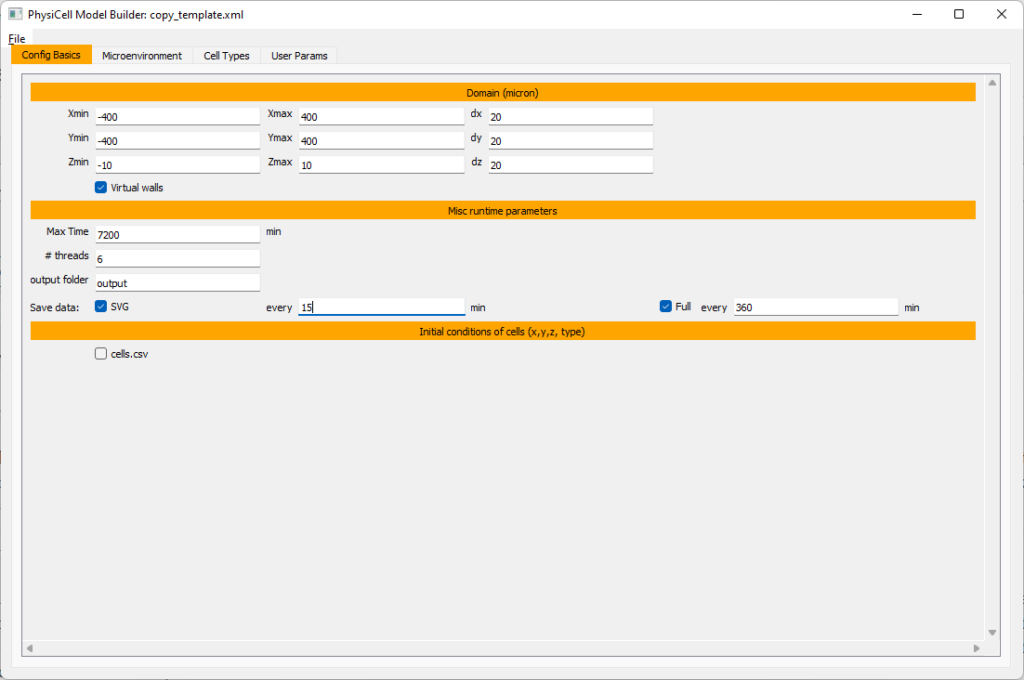#### Setting up diffusing substrates

Move to the microenvironment tab. Click on substrate and rename it to tumor signal . Set the decay rate to 0.1 and diffusion parameter to 10000. Uncheck the Dirichlet condition box so that it’s a zero-flux (Neumann) boundary condition.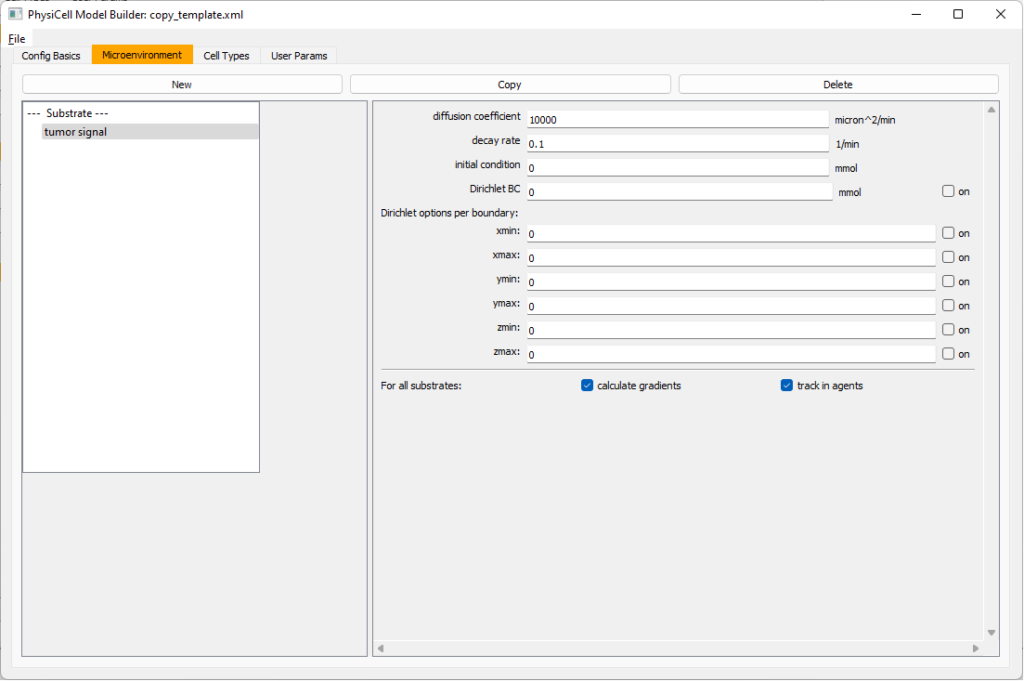Now, select tumor signal in the lefthand column, click copy, and rename the new substrate (substrate01) to pro-inflammatory signal with the same parameters.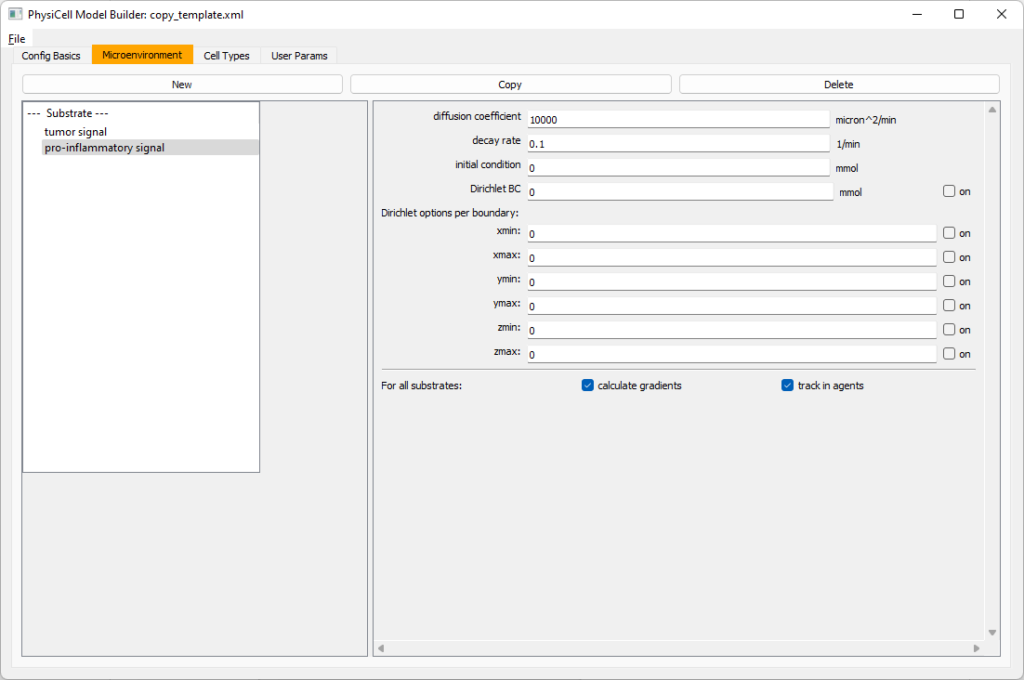Then, copy this substrate one more time, and rename it from name debris. Set its decay rate to 0.01, and its diffusion parameter to 1.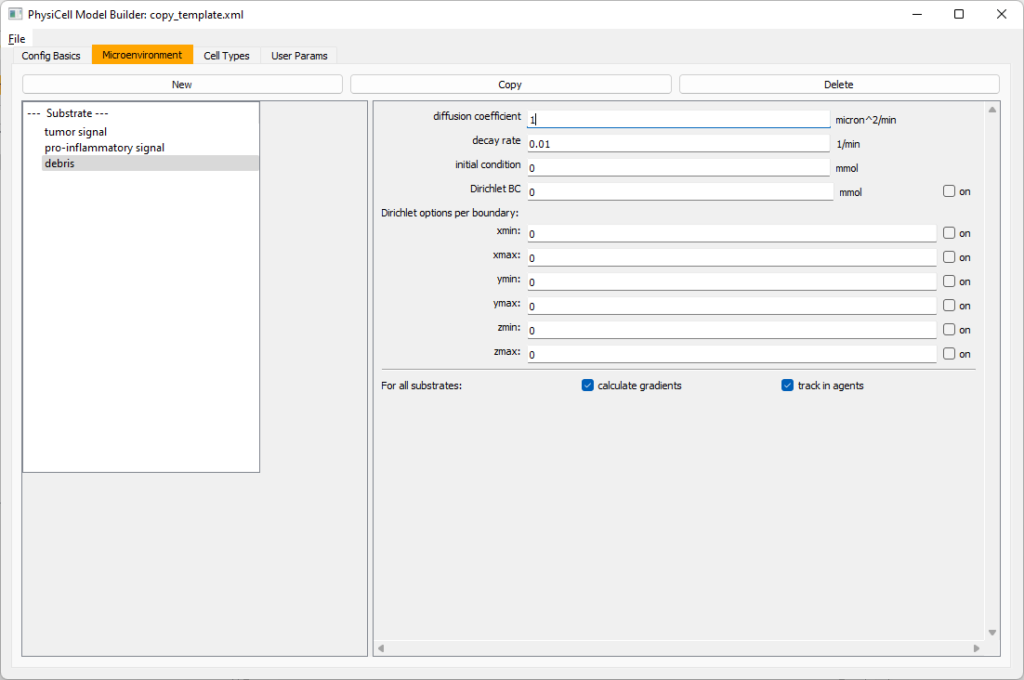Let’s save our work before continuing. Go to File, Save as, and save it as PhysiCell_settings.xml in your config file. (Go ahead and overwrite.)

If model builder crashes, you can reopen it, then go to File, Open, and select this file to continue editing.

#### Setting up the WT cancer cells

Move to the cell types tab. Click on the default cell type on the left side, and rename it to WT cancer cell. Leave most of the parameter values at their defaults. Move to the secretion sub-tab (in phenotype). From the drop-down menu, select tumor signal. Let’s set a high secretion rate of 10.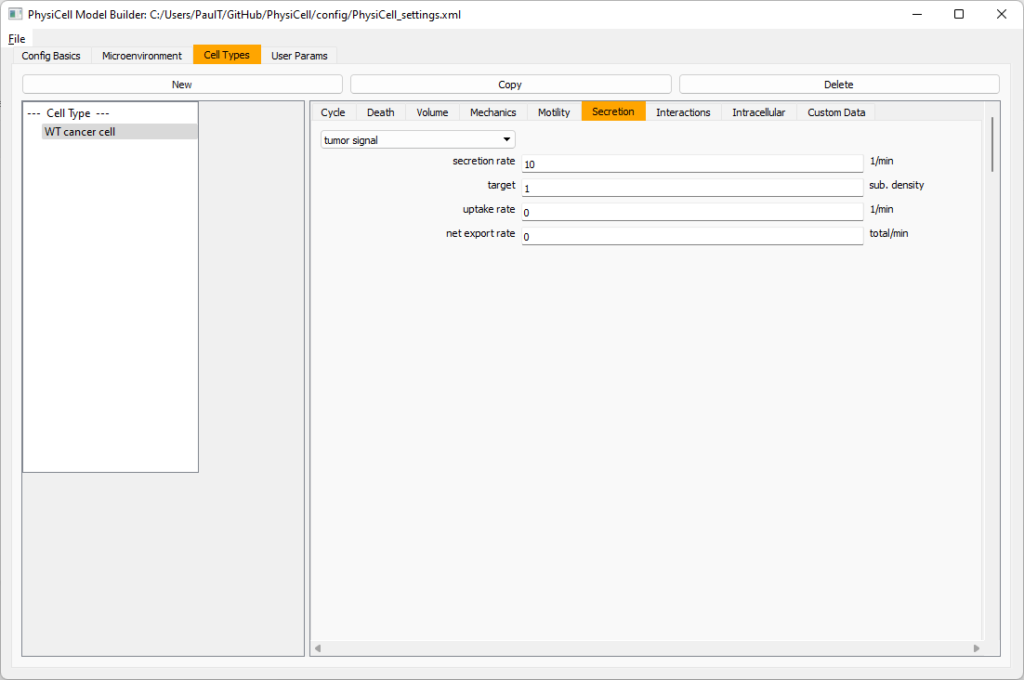We’ll need to set WT cells as able to mutate, but before that, the model builder needs to “know” about that cell type. So, let’s move on to the next cell type for now.

Setting up mutant tumor cells

Click the WT cancer cell in the left column, and click copy. Rename this cell type (for me, it’s cell_def03) to mutant cancer cell.  Click the secretion sub-tab, and set its secretion rate of tumor signal lower to 0.1.

#### Set the WT mutation rate

Now that both cell types are in the simulation, we can set up mutation. Let’s suppose the mean time to a mutation is 10000 minutes, or a rate of 0.0001 min$$^{-1}$$.

Click the WT cancer cell in the left column to select it. Go to the interactions tab. Go down to transformation rate, and choose mutant cancer cell from the drop-down menu. Set its rate to 0.0001.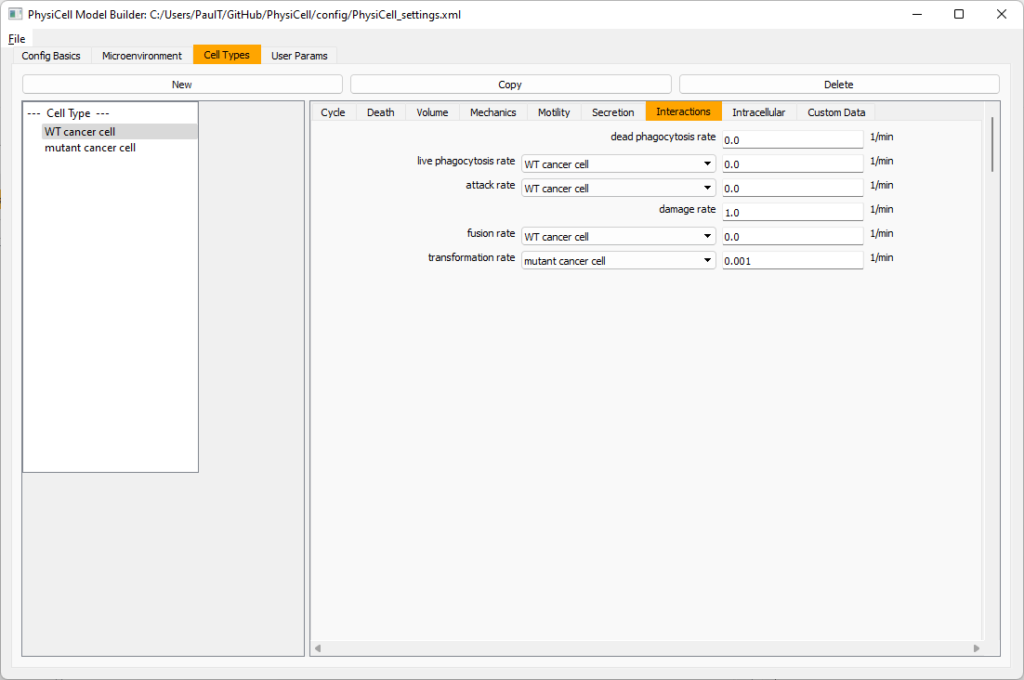#### Create macrophages

Now click on the WT cancer cell type in the left column, and click copy to create a new cell type. Click on the new type (for me cell_def04) and rename it to macrophage.

Click on cycle and change to a live cells and change from a duration representation to a transition rate representation. Keep its cycling rate at 0.

Go to death and change from duration to transition rate for both apoptosis and necrosis, and keep the death rates at 0.0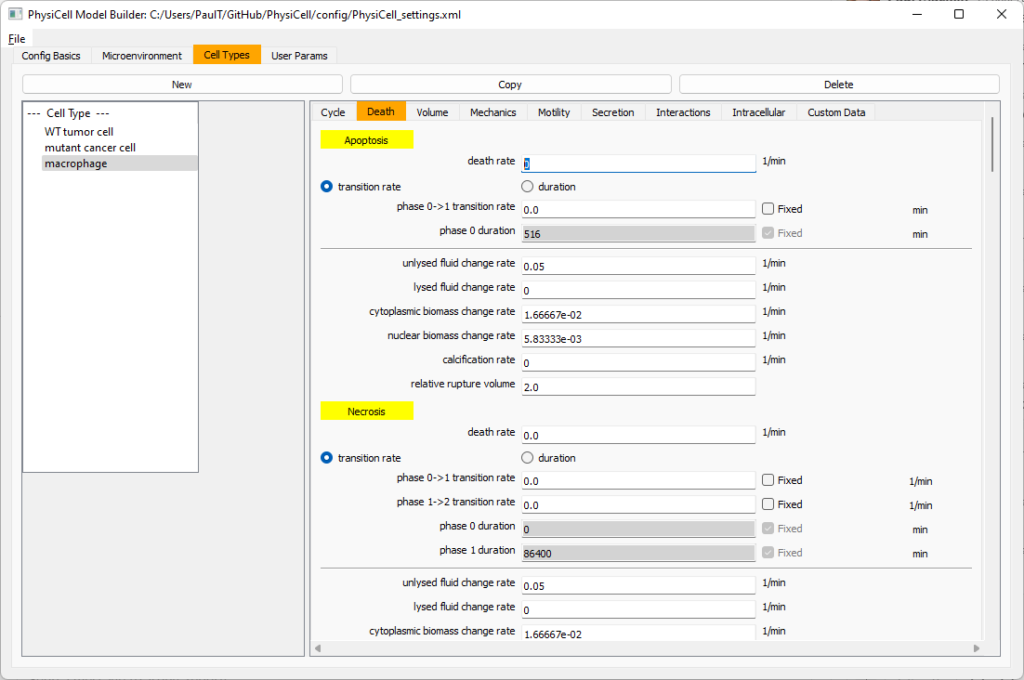Now, go to the motility tab. Set the speed to 1 $$\mu m/$$min, persistence time to 5 min, and migration bias to 0.5. Be sure to enable motility. Then, enable advanced motility. Choose tumor signal from the drop-down and set its sensitivity to 1.0. Then choose debris from the drop-down and set its sensitivity to 0.1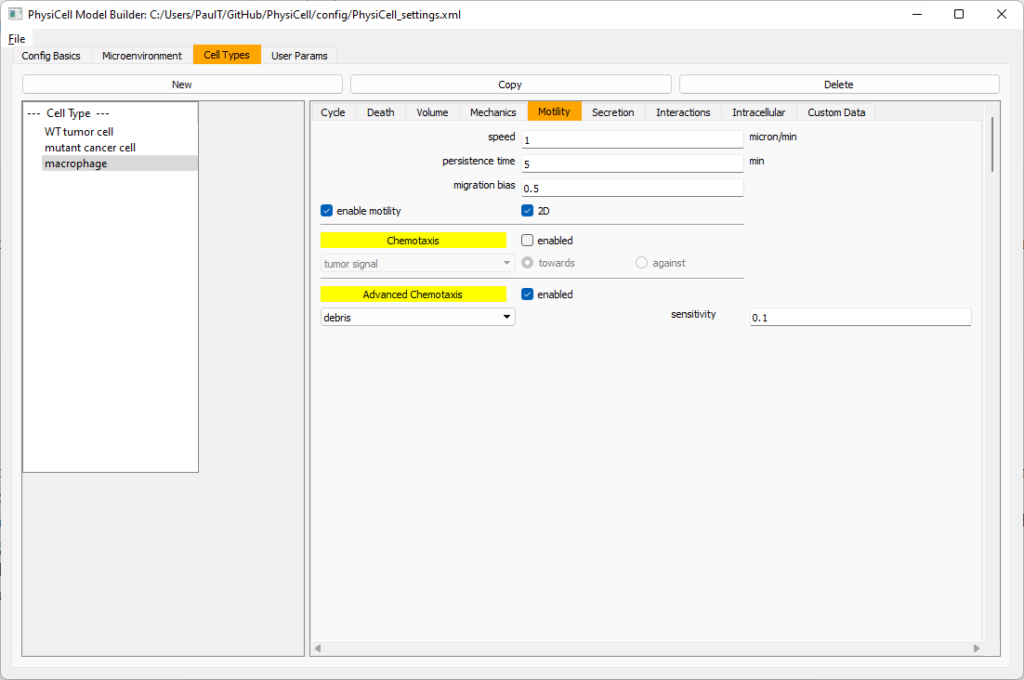Now, let’s make sure it secretes pro-inflammatory signal. Go to the secretion tab. Choose tumor signal from the drop-down, and make sure its secretion rate is 0. Then choose pro-inflammatory signal from the drop-down, set its secretion rate to 100, and its target value to 1.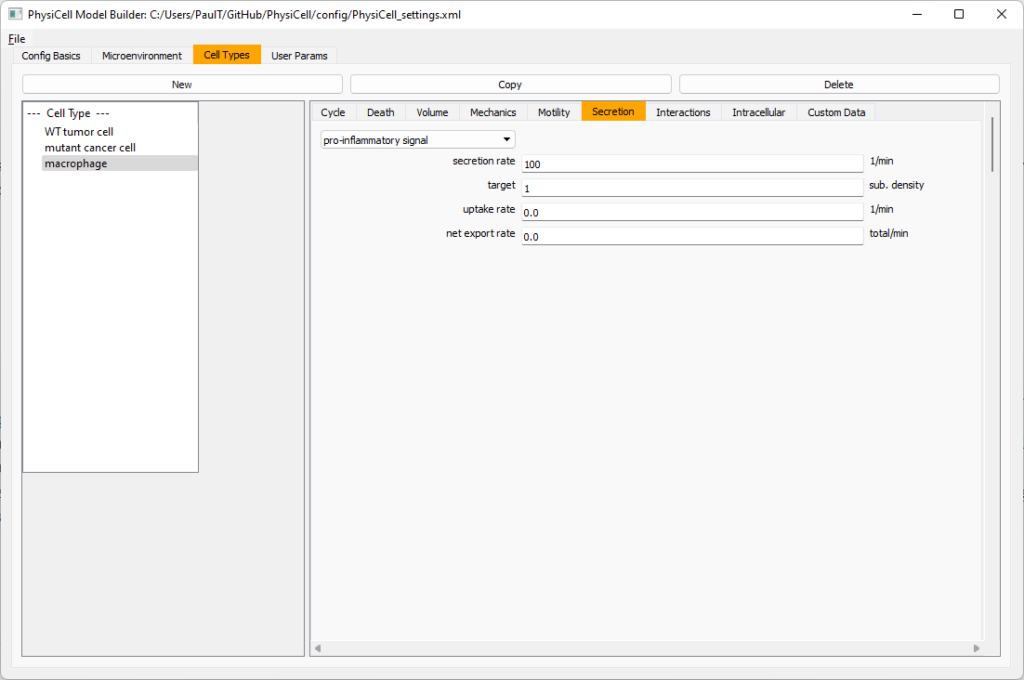Lastly, let’s set macrophages up to phagocytose dead cells. Go to the interactions tab. Set the dead phagocytosis rate to 0.05 (so that the mean contact time to wait to eat a dead cell is 1/0.05 = 20 minutes). This would be a good time to save your work.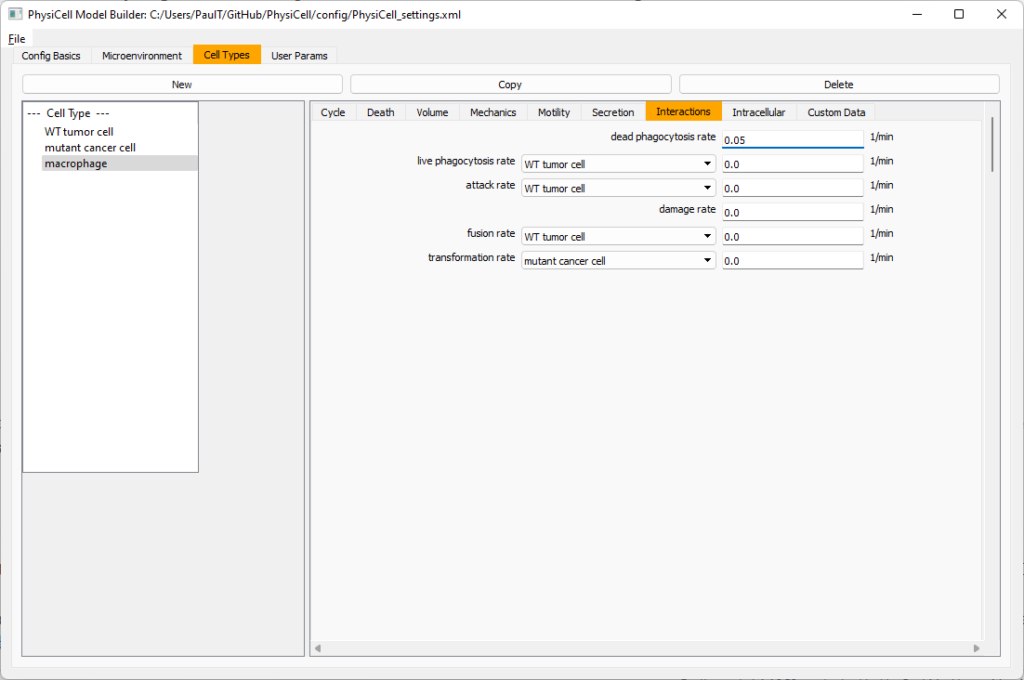Macrophages probably shouldn’t be adhesive. So go to the mechanics sub-tab, and set the cell-cell adhesion strength to 0.0.

#### Create CD8+ T cells

Select macrophage on the left column, and choose copy. Rename the new cell type to CD8+ T cell.  Go to the secretion sub-tab and make sure to set the secretion rate of pro-inflammatory signal to 0.0.

Now, let’s use simpler chemotaxis for these towards pro-inflammatory signal. Go to the motility tab. Uncheck advanced chemotaxis and check chemotaxis. Choose pro-inflammatory signal from the drop-down, with the towards option.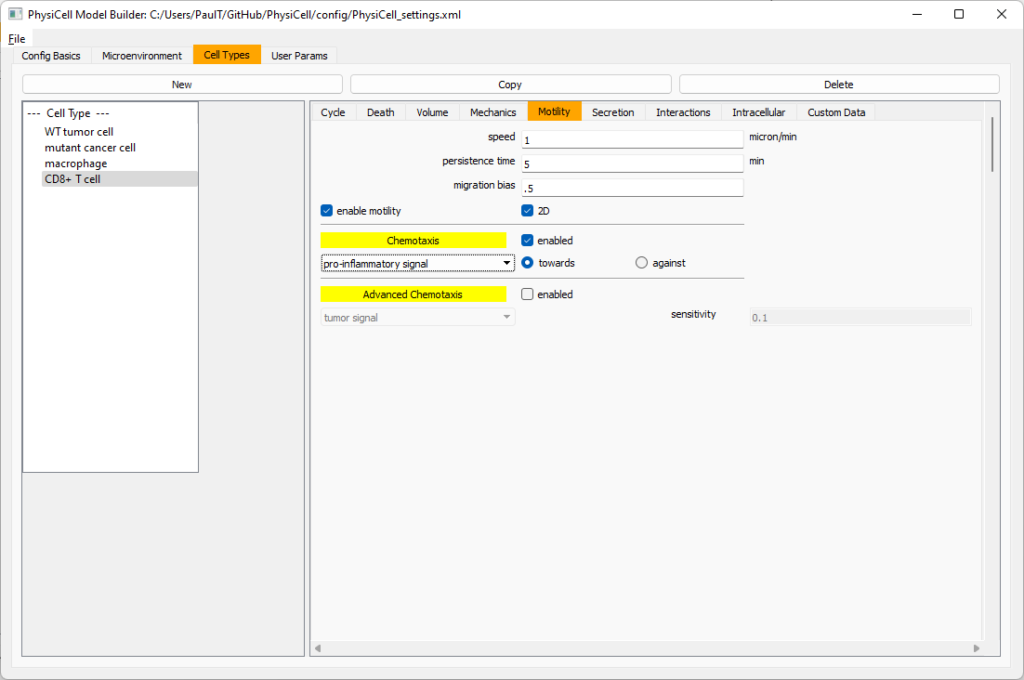Lastly, let’s set these cells to attack WT and tumor cells. Go to the interactions tab. Set the dead phagocytosis rate to 0.

Now, let’s work on attack. Set the damage rate to 1.0. Near attack rate, choose WT tumor cell from the drop-down, and set its attack rate to 10 (so it attacks during any 0.1 minute interval). Then choose mutant tumor cell from the drop-down, and set its attack rate to 1 (as a model of immune evasion, where they are less likely to attack a non-WT tumor cell).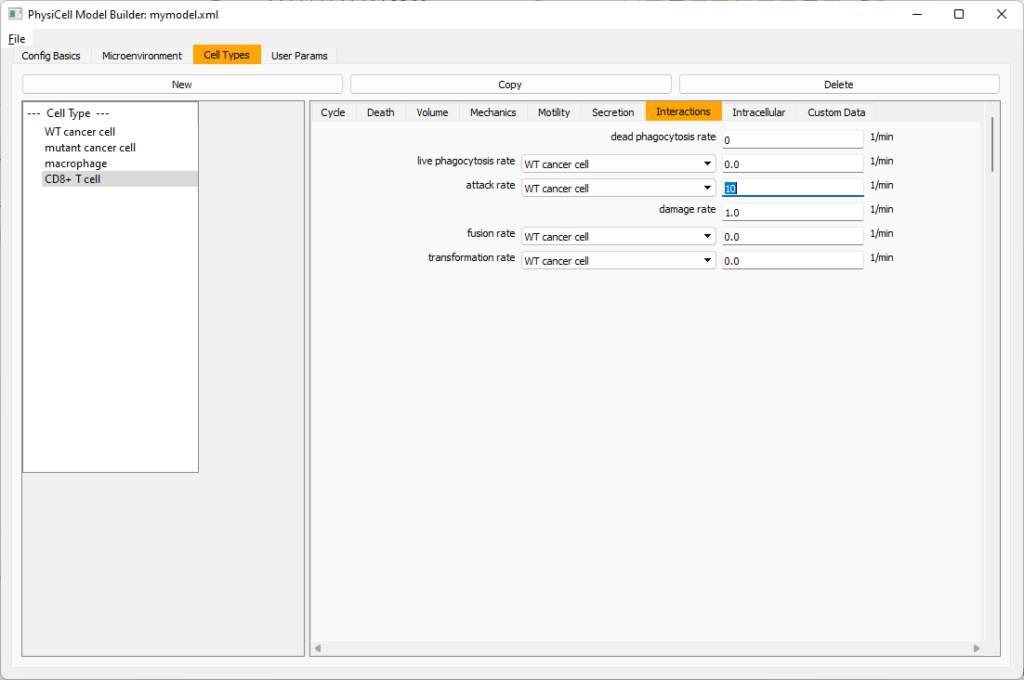#### Set the initial cell conditions

Go to the user params tab. On number of cells, choose 50. It will randomly place 50 of each cell type at the start of the simulation.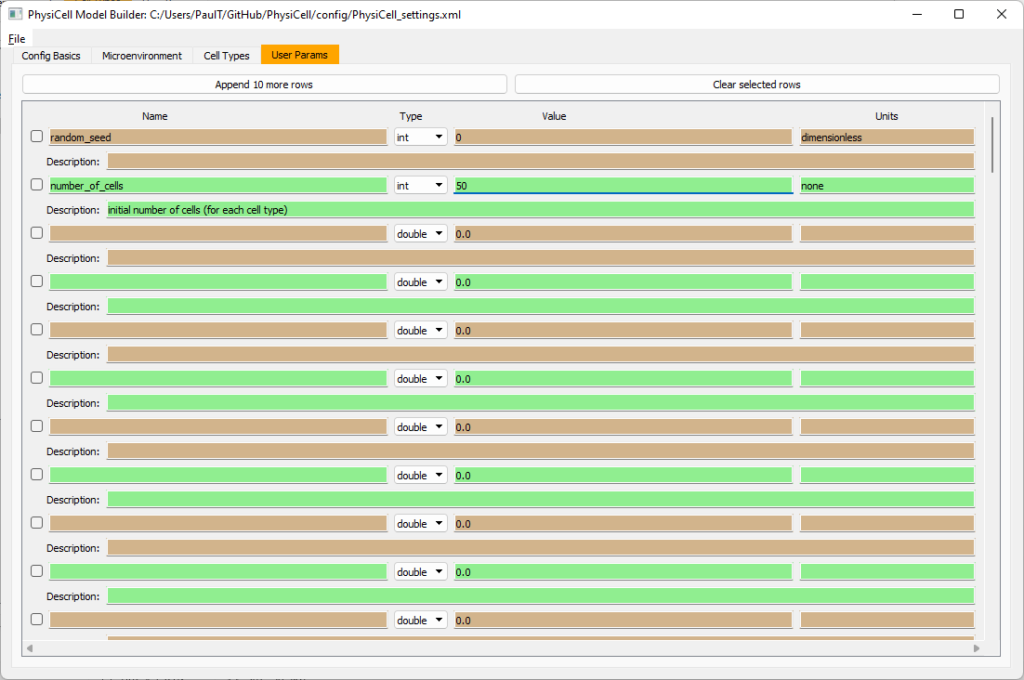That’s all! Go to File and save (or save as), and overwrite config/PhysiCell_settings.xml. Then close the model builder.

#### Testing the model

Go into the PhysiCell directory. Since you already compiled, just run your model (which will use PhysiCell_settings.xml that you just saved to the config directory). For Linux or MacOS (or similar Unix-like systems):

./project


Windows users would type project without the ./ at the front.

Take a look at your SVG outputs in the output directory. You might consider making an animated gif (make gif) or a movie:

make jpeg && make movie


Here’s what we have.

Promising! But we still need to write a little C++ so that damage kills tumor cells, and so that dead tumor cells release debris. (And we could stand to “anchor” tumor cells to ECM so they don’t get pushed around by the immune cells, but that’s a post for another day!)

#### Customizing the WT and mutant tumor cells with phenotype functions

First, open custom.h in the custom_modules directory, and declare a function for tumor phenotype:

void tumor_phenotype_function( Cell* pCell, Phenotype& phenotype, double dt );


Now, let’s open custom.cpp to write this function at the bottom. Here’s what we’ll do:

• If dead, set rate of debris release to 1.0 and return.
• Otherwise, we’ll set damage-based apoptosis.
• Get the base apoptosis rate
• Get the current damage
• Use a Hill response function with a half-max of 36, max apoptosis rate of 0.05 min$$^{-1}$$, and a hill power of 2.0

Here’s the code:

void tumor_phenotype_function( Cell* pCell, Phenotype& phenotype, double dt )
{
// find my cell definition
static Cell_Definition* pCD = find_cell_definition( pCell->type_name );

// find the index of debris in the environment
static int nDebris = microenvironment.find_density_index( "debris" );
static int nTS = microenvironment.find_density_index( "tumor signal" );

// if dead: release debris. stop releasing tumor signal
{
phenotype.secretion.secretion_rates[nDebris] = 1;
phenotype.secretion.saturation_densities[nDebris] = 1;

phenotype.secretion.secretion_rates[nTS] = 0;

// last time I'll execute this function (special optional trick)
pCell->functions.update_phenotype = NULL;
return;
}

// damage increases death

// find death model
static int nApoptosis =
phenotype.death.find_death_model_index( PhysiCell_constants::apoptosis_death_model );

double signal = pCell->state.damage; // current damage
double base_val = pCD->phenotype.death.rates[nApoptosis]; // base death rate (from cell def)
double max_val = 0.05; // max death rate (at large damage)

static double damage_halfmax = 36.0;
double hill_power = 2.0;

// evaluate Hill response function
double hill = Hill_response_function( signal , damage_halfmax , hill_power );
// set "dose-dependent" death rate
phenotype.death.rates[nApoptosis] = base_val + (max_val-base_val)*hill;

return;
}


Now, we need to make sure we apply these functions to tumor cell. Go to create_cell_types and look just before we display the cell definitions. We need to:

• Search for the WT tumor cell definition
• Set the update_phenotype function for that type to the tumor_phenotype_function we just wrote
• Repeat for the mutant tumor cell type.

Here’s the code:

...
/*
Put any modifications to individual cell definitions here.

This is a good place to set custom functions.
*/

cell_defaults.functions.update_phenotype = phenotype_function;
cell_defaults.functions.custom_cell_rule = custom_function;
cell_defaults.functions.contact_function = contact_function;

Cell_Definition* pCD = find_cell_definition( "WT cancer cell");
pCD->functions.update_phenotype = tumor_phenotype_function;

pCD = find_cell_definition( "mutant cancer cell");
pCD->functions.update_phenotype = tumor_phenotype_function;

/*
This builds the map of cell definitions and summarizes the setup.
*/

display_cell_definitions( std::cout );
return;
}


That’s it! Let’s recompile and run!

make && ./project


And here’s the final movie.

Much better! Now the

### Coming up next!

We will use the signal and behavior dictionaries to help us easily write C++ functions to modulate the tumor and immune cell behaviors.

Once ready, this will be posted at http://www.mathcancer.org/blog/introducing-cell-signal-and-behavior-dictionaries/.

Tags :